SSAT Upper Level Math : How to find decimal fractions

Example Questions

2 Next →

Example Question #11 : How To Find Decimal Fractions

Convert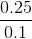to a fraction.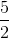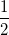Explanation:

Lets try to get these values into whole numbers. To do that, we need to make sure both numerator and denominator are whole numbers and we will look for the number with the most decimal places. This is found in the numerator. To get it into a whole number, we will multiply byor move the decimal placesto the right. When you do that for the numerator, the same applies for the denominator. The new fraction is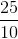. Just reduce the fraction by factoring out aand the answer is shown.

Example Question #12 : How To Find Decimal Fractions

What's the answer in decimal form?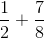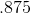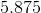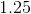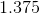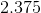Explanation:

Convert each fraction to decimal. We haveand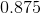. Add these values. Remember when adding decimals, make sure the decimal places are all lined up.

Example Question #13 : How To Find Decimal Fractions

Convert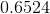into a simplified fraction.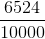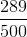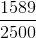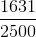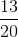Explanation:

Lets place aunder the decimal. We are going to make a fraction. Essentially, it's still the same value. Now, we want a whole number in the numerator and what we do to the numerator is done to the denominator as well. Lets multiply the top by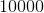or move the decimal placespots to the right. Now we have. This needs to be reduced, so divide bytwice until it can't be reduced any further. The answer should beExample Question #14 : How To Find Decimal Fractions

Convert to a fraction.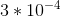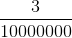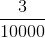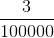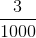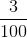Explanation:

First, lets convert the exponent into a fraction. Any negative exponent means it's the reciprocal of the positive exponent. So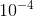means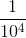. Now lets multiply it with the. This means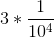or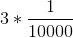or.

Example Question #15 : How To Find Decimal Fractions

Convert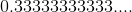into a fraction.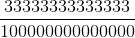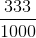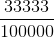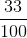Explanation:

Letbe.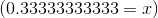Lets multiplyby. Now we have: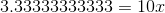Lets subtract this equation with the first one and we get: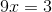We do this because we want to get rid of the repeating decimals and now we have a simple equation, isolateand we arrive at the final answer.

Example Question #16 : How To Find Decimal Fractions

Convert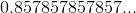into a fraction.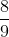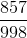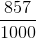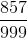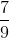Explanation:

Let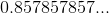be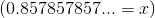Lets multiplyby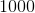. I chose, because there is a set ofnumbers that make the repeating decimal. To determine the value to multiply the repeating decimal, we doto the power of number in a set before it repeats.

Now we have: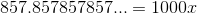Lets subtract this equation with the first one and we get: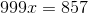We do this because we want to get rid of the repeating decimals and now we have a simple equation, isolateand we arrive at the final answer.

2 Next →